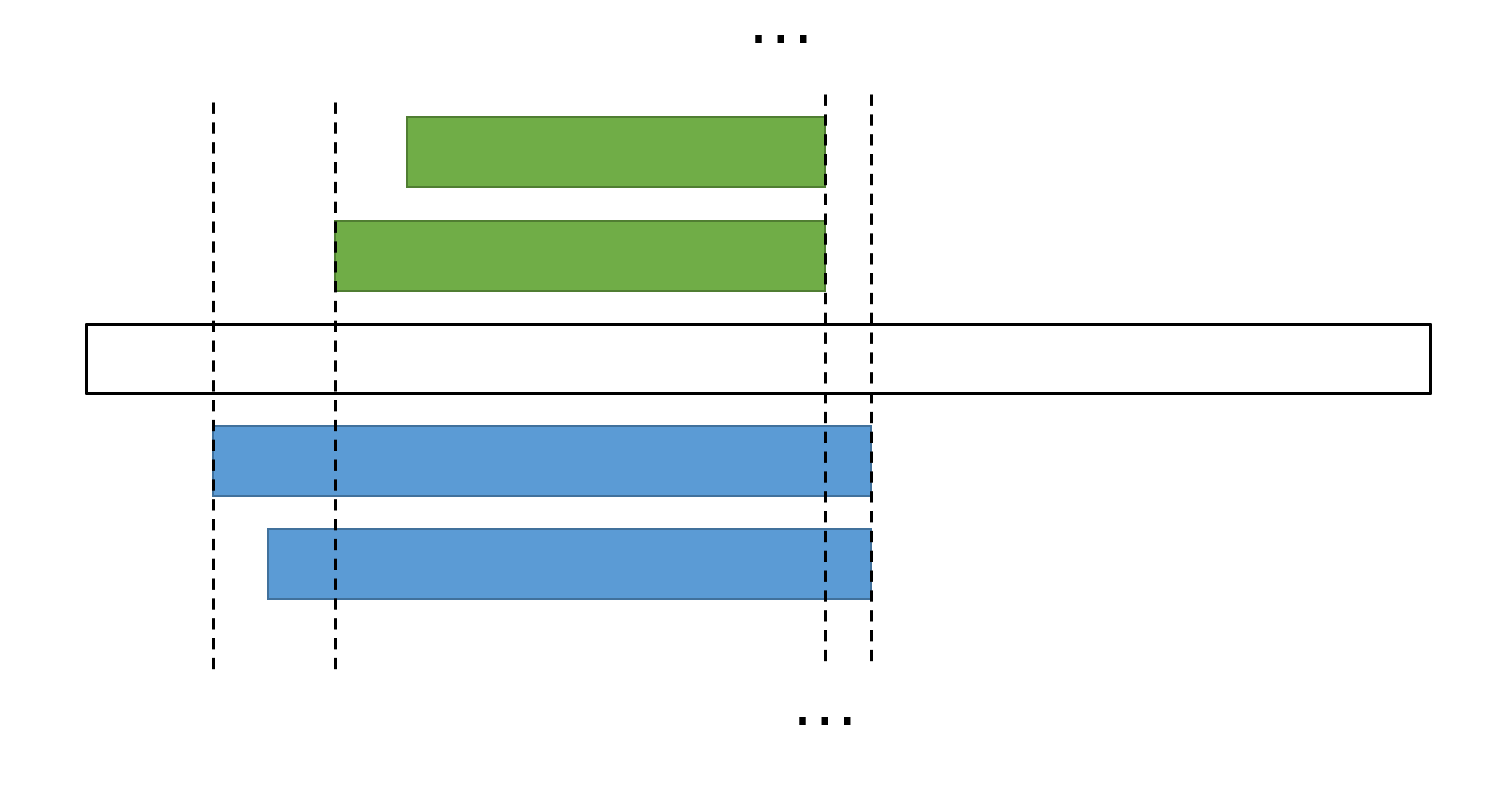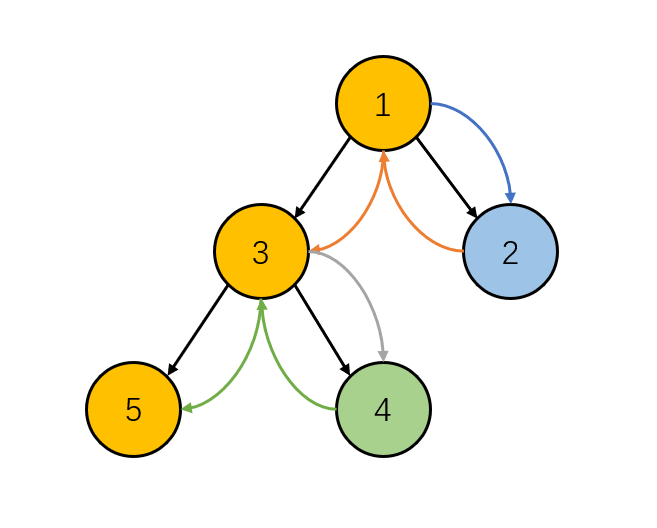# 题解

XLex 正则表达式

rank solved A B C D E F G H I J K L M
96 9 Ø . O Ø Ø O O . O . O . O

# F Examrank solved A B C D E F G H I J K
7 6 O . . O O O O . . . O

# 分析# 分析

$$\begin{eqnarray} g(k) & = & \sum_{i = k}^n a_i \frac{i!}{k!(i-k)!} \ g(k) & = & \frac{1}{k!} \sum_{i = k}^{n} (a_i \cdot i!) \times \frac{1}{(i-k)!} \end{eqnarray}$$